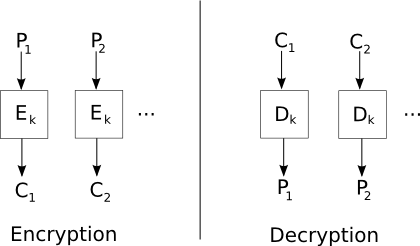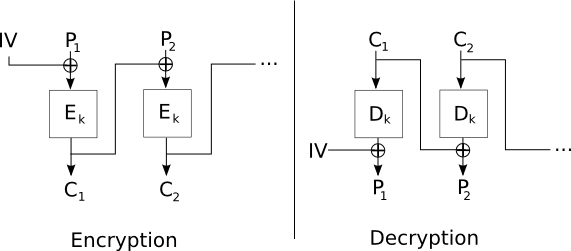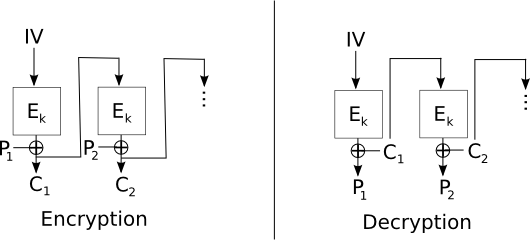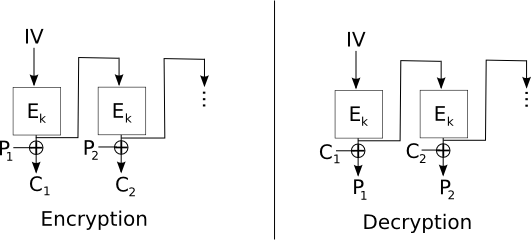# Symmetric-Key Cryptography

Lecturer: Tom Roeder
Lecture notes by Tom Roeder

## 1 One-key operations: Symmetric Cryptography

A Message Authentication Code (MAC) is a keyed scheme that provides authentication, like a signature, but only between two hosts. A MAC takes a key k and a message m and produces a tag t = MAC(m, k) such that it is hard for anyone that does not know k to produce a tag t' and message m' such that t' = MAC(m', k).

One well-studied and popular MAC, called HMAC, uses hash functions to compute a MAC. It is constructed as follows, where || represents concatenation:

HMAC(m, k) = h( (k XOR opad) || h( (k XOR ipad) || m) )

where opad = (01011100) and ipad = (00110110). The values of opad and ipad were carefully chosen to ensure that each input bit of the message affects all the bits of the output.

A MAC is an instance of a one-key primitive built on a zero-key primitive. MACs achieve integrity. A major goal of one-key or symmetric cryptography primitives, however, is to enable confidential communication between two parties.

### 1.1 High level and history

#### 1.1.1 Motivation: Confidential Communication

Alice and Bob are spending their last few moments together before being separated. How can they pass information confidentially once they're separated? One idea would be to share a key now that they could later use to encode their communication. Confidential communication is one of the original motivating problems in cryptography. Early techniques for confidential communication used simple permutations and letter-rearranging games, but the 20th century saw cryptography move squarely into the domain of mathematics.

#### 1.1.2 DES and modern cryptography

Much of the development of modern cryptography was spurred on by the acceptance, in 1976 of an algorithm from IBM (with collaboration by the NSA) that became the Data Encryption Standard (DES), a federal standard for shared-key encryption. Cryptographers at the time worried that the NSA had modified the algorithm to make it weaker, reducing the effective key length to 56 bits from 64 bits and modifying some of the internal structures. A great deal of research in the ensuing decades went into cryptanalysis of DES and related schemes. In the early 90's, however, the (public) discovery of differential cryptanalysis made it clear that no structural weaknesses had been introduced. In fact, differential cryptanalysis of DES revealed that IBM and the NSA knew about differential cryptanalysis 20 years earlier, since internal DES structures were much more resistant to this form of attack than they would have been if they had been chosen at random.

The secretive process by which DES was chosen and modified was a major cause of concern and distrust in the cryptographic community. More recently, the Advanced Encryption Standard (AES) was chosen as a replacement for DES via a much improved and entirely public process of proposals and cryptanalysis.

### 1.2 Definitions for Encryption

To define shared-key encryption, we first assume that a key is shared between two principals. Later lectures will show how to discharge this sharing obligation under different setup assumptions.

For our purposes, an encryption scheme consists of two functions, an Encryption function E that takes a key and a message (known as the plaintext) and outputs an encoded message (known as the ciphertext), and a decryption function D that takes a key and a ciphertext and outputs plaintext. When there is no possibility of confusion about the encryption function being used, a message m encrypted under a key k is written {m}k. Two main properties should hold for an encryption function.

1. (Completeness) Given any message m and key k, encrypted messages can always be decrypted: Dk(Ek(m)) = m.
2. (Semantic Security) Loosely speaking, this property requires that it is hard to invert an encryption function without knowing the key. To state this property more formally requires a notion of the appropriate attack model: an adversary that attempts to break the scheme might have various sources of information.
• Chosen Plaintext Attack (CPA)

In the simplest attack model, known as Chosen Plaintext Attack (CPA), the adversary has access to a machine that will perform arbitrary encryptions but will not reveal the shared key. This machine corresponds intuitively to being able to see many encryptions of many messages before trying to decrypt a new message.

In this case, Semantic Security requires that it be computationally hard for any adversary to distinguish an encryption Ek(m) from Ek(m') for two arbitrarily chosen messages m and m'. Distinguishing these encryptions should be hard even if the adversary can request encryptions of arbitrary messages.

Note that this property cannot be satisfied if the encryption function is deterministic! In this case, the adversary can simply request an encryption of m and an encryption of m' and compare them. This is a point that you should all remember when implementing systems: encrypting under a deterministic function with no randomness in the input does not provide Semantic Security.

For example, a general wishing to send the message "attack" would want to ensure that no adversary receiving this message could distinguish from any other message, such as "retreat".

• Chosen Ciphertext Attack (CCA)

Under the CCA model, an adversary has access to an encryption and a decryption machine and must perform the same task of distinguishing encryptions of two messages of its choice. First, the adversary is allowed to interact with the encryption and decryption services and choose the pair of messages. After it has chosen the messages, however, it only has access to an encryption machine.

This attack model is often called the "lunchtime" attack, by analogy with an adversary that sneaks into your office to use your computer while you're away at lunch (thus getting access to an encryption and decryption machine); this adversary must later succeed at analyzing a new message.

• Chosen Ciphertext Attack 2 (CCA2)

CCA2 security has the same model as CCA security, except that the adversary retains access to the decryption machine after choosing the two messages. To keep this property from being trivially violated, we require that the adversary not be able to decrypt the ciphertext it is given to analyze.

This attack model is often called the "midnight" attack, by analogy with a lunchtime attacker that sneaks back in at midnight after choosing messages and is able to use your computer again.

Semantic Security can only be achieved under probabilistic encryption schemes, but most common schemes are deterministic. One way to get a probabilistic scheme from deterministic scheme is to concatenate a random string to the message before encrypting: E'k(m) = Ek(m || r). Then decryption simply removes the random string: D'k(m || r) = m.

### 1.3 Nonces

A nonce is a bit string that satisfies Uniqueness (also known as Freshness), which means that it has not occurred before in a given run of a protocol. Nonces might also satisfy Unpredictability, which effectively requires pseudo-randomness: no adversary can predict the next nonce that will be chosen by any principal. There are several common sources of nonces.

• Counter

Counters are the simplest nonces to implement, but they require that principals keep the state of the counter. Further, although they often trivially satisfy Uniqueness for a given principal, they never satisfy Unpredictability. In some protocols, Unpredictability is not necessary.

• Time

The main advantage of time as a nonce over counters is that most machines already keep track of some notion of time, so there is no need to explicitly track state.

• Random numbers

To ensure that that truly random numbers satisfy Uniqueness perfectly, it would be necessary to keep a large amount of state. But if the space of random numbers is large enough, random choice usually gives a small enough probability of collisions to guarantee that the properties of a given system will be satisfied. This is the only source of nonces that satisfies Unpredictability (of course, PRFs could be used, but this scheme still must keep track of all previous outputs to guarantee Uniqueness perfectly).

Given the attack models and definitions of encryption shown above, it may seem that encryption schemes must be very complex to construct. Although there are many complex and useful encryption schemes, there is at least one scheme that is provably, perfectly secure. It just happens not to be practical in most contexts. This scheme is called One-Time Pad (OTP) encryption and was proven to be secure by Shannon in 1949. The functions are computed as follows: A and B agree on a random number k that is as long as the message they later want to send.

• Ek(x) = x XOR k
• Dk(x) = x XOR k

Note that since k is chosen at random and not known to an adversary, the output of this scheme is indistinguishable to an adversary from a random number. But it suffers from several drawbacks.

• Not CPA secure: suppose that an adversary can request encryptions. The adversary requests the encryption of a block 00...0 (the length of the key) and gets Ek(00...0) = 00...0 XOR k, so the adversary can read off the value of k.

We can get around this problem using a pseudo-random function on a random input to build up a one-time pad and XOR it against a message. To do so, start with a random initialization vector iv and a key k for the PRF. Compute fk(iv) = x1 and output the first block c1 = x1 XOR p1. For block i, compute fk(xi-1) = xi and output the ith block as ci = xi XOR pi. This scheme illustrates how to extend a random iv to a long value suitable for use in schemes similar to OTP encryption.

• Malleable: An encryption scheme is said to satisfy Non-Malleability if it is computationally hard for an adversary to compute the encryption of any non-trivial function of an encrypted message (CCA2 security can be shown to imply Non-Malleability). Unfortunately, it is easy to modify this encrypted value to be an encryption of the same value plus or minus one. Set m' = 00..01 (a bit string of the same length but with a one at the end), take Ek(m) = k XOR m, and compute Ek(m) XOR m' = k XOR m XOR m' = Ek(m XOR m').

A classic application for which Non-Malleability is required is in an auction; bidders would prefer to be hard for other bidders to use their encrypted bids to produce bids that are, say, \$1 higher.

### 1.5 Types and Modes of Encryption

Encryption functions normally take a fixed-size input to a fixed-size output, so encryption of longer units of data must be done in one of two ways: either a block is encrypted at a time and the blocks are somehow joined together to make the ciphertext, or a longer key is generated from a shorter one and XOR'd against the plaintext to make the ciphertext. Schemes of the former type are called block ciphers, and schemes of the latter type are called stream ciphers.

#### 1.5.1 Block ciphers

Block ciphers take as input the key and a block, often the same size as the key. Further, the first block is often augmented by a block called the initialization vector, which can add some randomness to the encryption.

• Electronic Code Book (ECB) mode
ECB mode divides the plaintext into blocks m1, m2, ..., mn, and computes the ciphertext ci = Ei(mi). This mode is vulnerable to many attacks and is not recommended for use in any protocols. Chief among its defects is its vulnerability to splicing attacks, in which encrypted blocks from one message are replaced with encrypted blocks from another.For example, if a protocol using the message {A, B, KAB}kA were encrypted in ECB mode, it might be possible to replace {A, B, KAB}kA with {A, B, KAT}kA using KAT from a message sent to A for communication with an adversary T. In this case, A would believe that it was communicating with B, but in fact all of its communication could be read by T.

Do not use ECB mode in your project!

• Cipher Block Chaining (CBC) mode
CBC mode remedies some of the problems of ECB mode by using an initialization vector and chaining the input of one encryption into the next. CBC mode starts with an initialization vector iv and XORs a value with the plaintext that is the input to each encryption. So, c1 = Ek(iv XOR m1) and ci = Ek(ci-1 XOR mi). If a unique iv is used, then no splicing attacks can be performed, since each block depends on all previous blocks along with the initialization vector.The iv is a good example of a nonce that needs to satisfy Uniqueness but not Unpredictability.

• Cipher Feed-Back (CFB) mode

CFB mode moves the XOR of CBC mode to the output of the encryption. In other words, c1 = Ek(iv) XOR m1, and ci = Ek(ci-1) XOR mi. This mode then suffers from failures of Non-Malleability, at least locally to every block, but changes to ciphertext do not propagate very far, since each block of ciphertext is used independently to XOR against a given block to get the plaintext.These failures can be seen in the following example, in which a message m = m1 m2 ... mn is divided into n blocks, and encrypted with an iv under CFB mode to c1 c2 ... cn. Suppose an adversary substitutes c'2 for c2. Then, in decryption, m1 = Ek(iv) XOR c1, which is correct, but m'2 = Ek(c1) XOR c'2, which means that m'2 = m2 XOR c2 XOR c'2, since m2 = Ek(c1) XOR c2. Thus, in m2, the adversary can flip any bits of its choice.

Then m'3 = Ek(c'2) XOR c3, which should lead to random looking message not under the adversary's control, since the encryption of c'2 should look random. But m4 = Ek(c3) XOR c4 and thereafter the decryption is correct.

• Output Feed-Back (OFB) mode

OFB mode modifies CFB mode to feed back the output of the encryption function to the encryption function without XOR-ing the ciphertext.#### 1.5.2 Stream ciphers

Unlike block ciphers, stream ciphers (such as RC4) produce a pseudo-random sequence of bits that are then combined with the message to give an encryption. Since the combining operation is often XOR, naive implementations of these schemes can be vulnerable to the sort of bit-flipping attacks on Non-Malleability that we have seen before.

Two types of stream ciphers exist: synchronous, in which state is kept by the encryption algorithm but is not correlated with the plaintext or ciphertext, and self-synchronizing, in which some information from the plaintext or ciphertext is used to inform the operation of the cipher.

#### 1.5.3 Degenerate Keys and IVs

Depending on the particular encryption scheme, some choices of keys and IVs are not recommended. For instance, it is never recommended to use a key as an initialization vector; some attacks can on block ciphers reveal the IV. Normally it is recommended that the IV be chosen randomly each time.

Similarly, some encryption schemes have a small number of weak keys that do not produce as random an output as encryption under other keys would. Cryptographic libraries normally provide key generation functions that avoid producing such keys.

### 1.6 Practical Examples

#### 1.6.1 DES

The history of DES was discussed above. It was the first encryption algorithm to be publicly certified by the NSA, and it stimulated great interest in block ciphers. DES runs 16 rounds of an iterated block cipher on a block size 64 with a 56-bit key (there are other bits in the key that are used for other purposes). DES is no longer secure; with modern hardware, the entire space of keys can be searched in short order.

An obvious simple improvement to DES would be to encrypt encryptions with a second key: 2DESk1, k2(m) = DESk1(DESk2(m)). 2DES turns out to be vulnerable to attacks called meet-in-the-middle, which reduces the security to the security of DES. So, the common replacement for DES is 3DES, also called TripleDES: 3DESk1, k2, k3 = DESk1(DESk2(DESk3(m))). TripleDES has an effective key length of 112 bits, well outside the range of current brute force attacks. But there is a new encryption standard that is recommended for use instead of DES.

#### 1.6.2 AES

The Advanced Encryption Standard (AES) was chosen in 2001 as the winner of a 5-year contest to replace the then outdated and insecure DES. AES is a version of the Rijndael algorithm designed by Joan Daemen and Vincent Rijmen. AES is also an iterated block cipher, with 10, 12, or 14 rounds for key sizes 128, 192, and 256 bits, respectively.

AES provides high performance symmetric key encryption and decryption. Although multitudes of cryptographers have examined it over the last 10 years or so, no substantial attacks against the algorithm itself have been published, so far.